Scale Factor A Look at Scale Factor and

• Slides: 15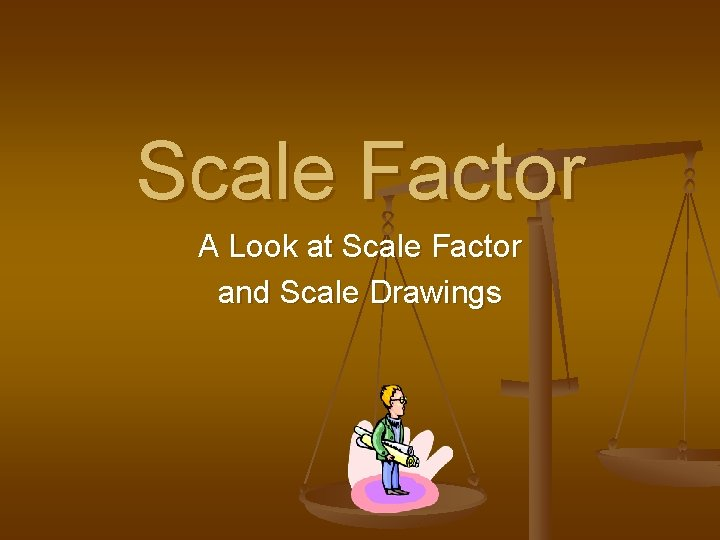Scale Factor A Look at Scale Factor and Scale Drawings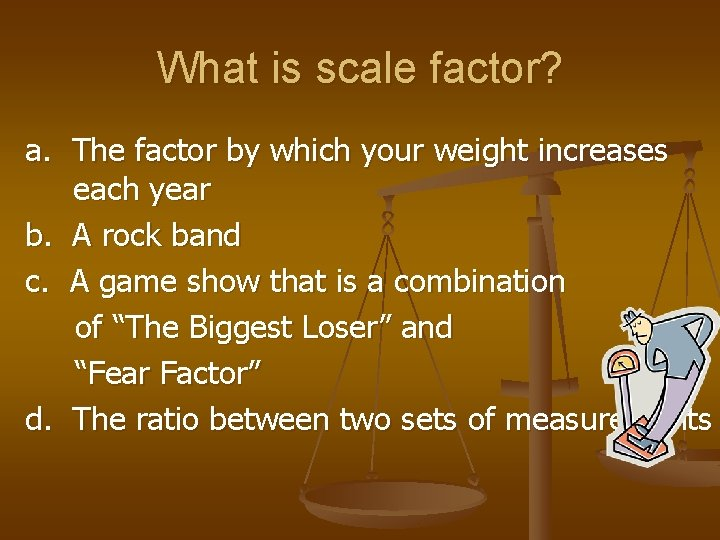What is scale factor? a. The factor by which your weight increases each year b. A rock band c. A game show that is a combination of “The Biggest Loser” and “Fear Factor” d. The ratio between two sets of measurementsThat’s right! Scale factor is the ratio between two sets of measurements. In a scale drawing, the scale factor is the ratio of the measurement on the drawing to the measurement of the actual object.For Instance… A blueprint is a scale drawing of a building. When you buy a home or look for an apartment, you will look at the blueprint. The scale factor on this is at the bottom. It says ¼” = 1’. What do you think this means?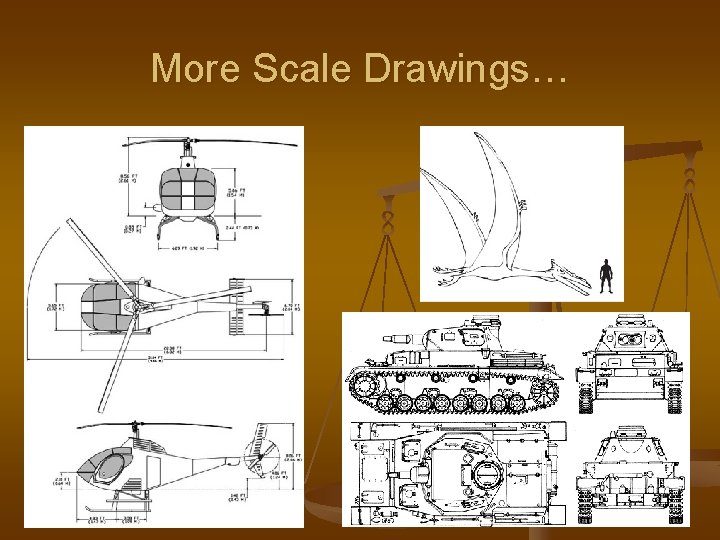More Scale Drawings…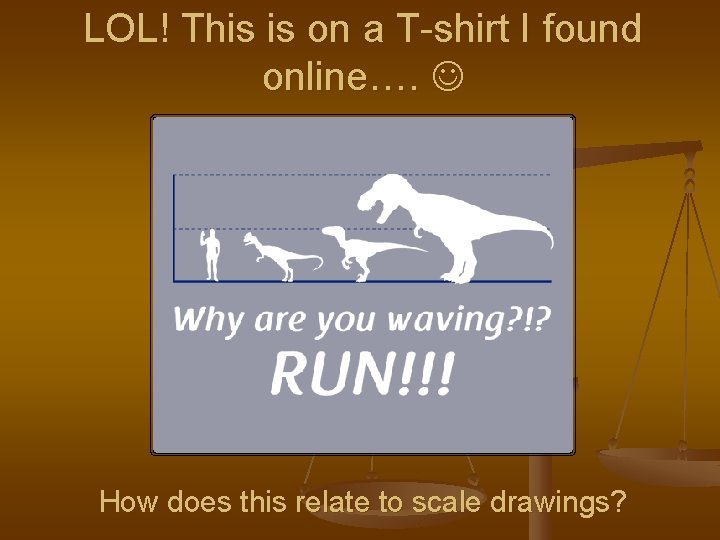LOL! This is on a T-shirt I found online…. How does this relate to scale drawings?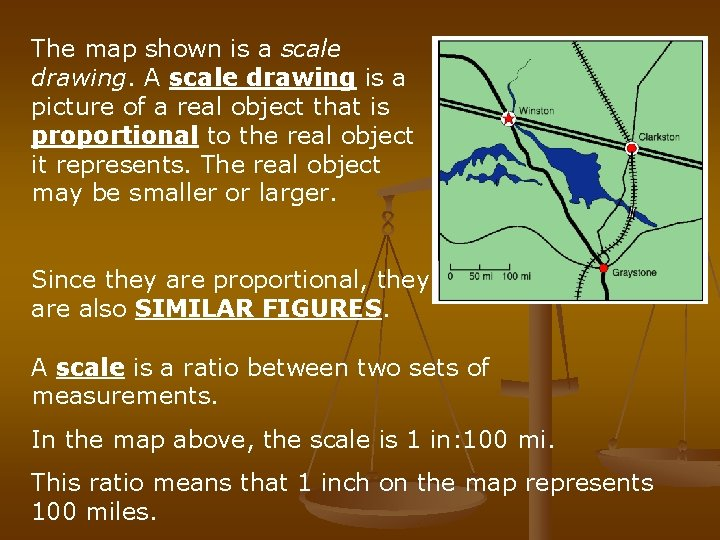The map shown is a scale drawing. A scale drawing is a picture of a real object that is proportional to the real object it represents. The real object may be smaller or larger. Since they are proportional, they are also SIMILAR FIGURES. A scale is a ratio between two sets of measurements. In the map above, the scale is 1 in: 100 mi. This ratio means that 1 inch on the map represents 100 miles.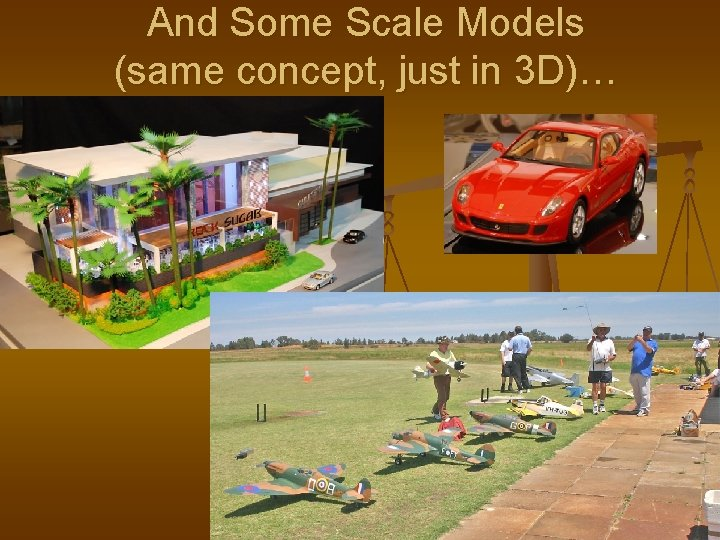And Some Scale Models (same concept, just in 3 D)…What do you Think? . . . Why are scale drawings and scale models useful? When might they be used in real life?One of the most common uses of scale factor is a map… 1 cm = 3 mi Notice that the key says 1 cm = 3 mi. What does that mean? Is it important to list this on a map? Why?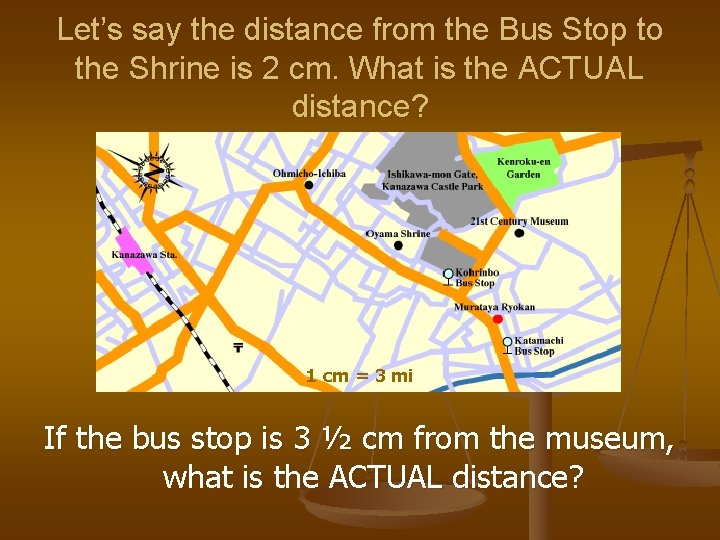Let’s say the distance from the Bus Stop to the Shrine is 2 cm. What is the ACTUAL distance? 1 cm = 3 mi If the bus stop is 3 ½ cm from the museum, what is the ACTUAL distance?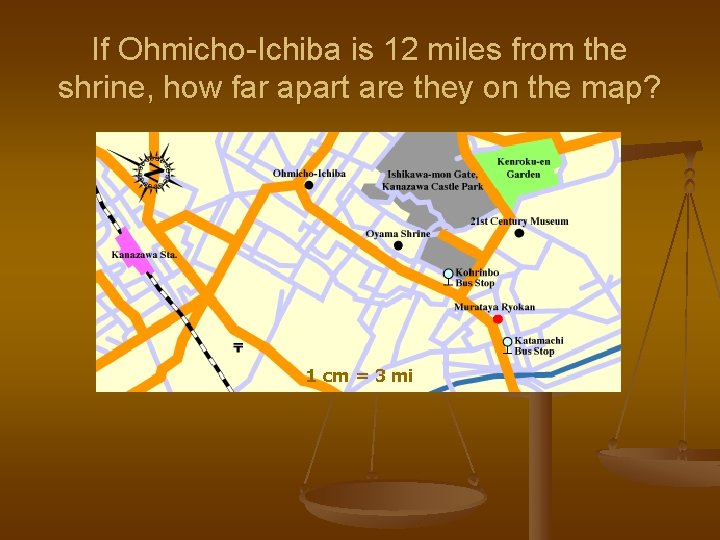If Ohmicho-Ichiba is 12 miles from the shrine, how far apart are they on the map? 1 cm = 3 mi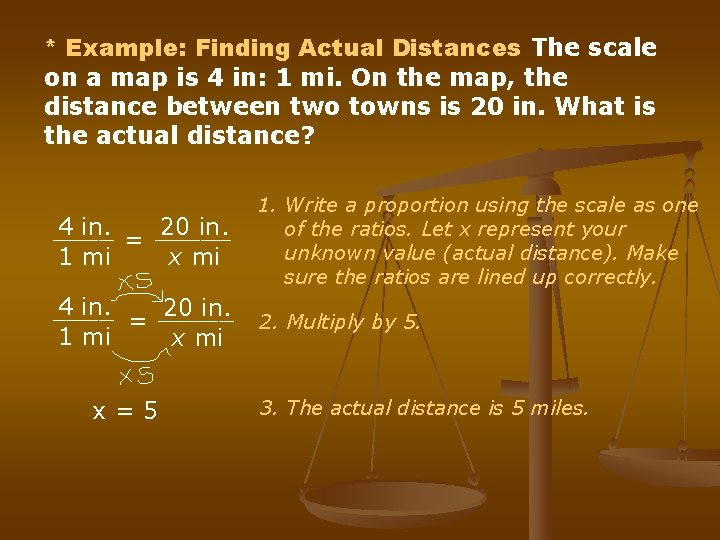* Example: Finding Actual Distances The scale on a map is 4 in: 1 mi. On the map, the distance between two towns is 20 in. What is the actual distance? 4 in. 20 in. ____ = _____ 1 mi x mi 1. Write a proportion using the scale as one of the ratios. Let x represent your unknown value (actual distance). Make sure the ratios are lined up correctly. 4 in. 20 in. ____ = _____ 1 mi x mi 2. Multiply by 5. x=5 3. The actual distance is 5 miles.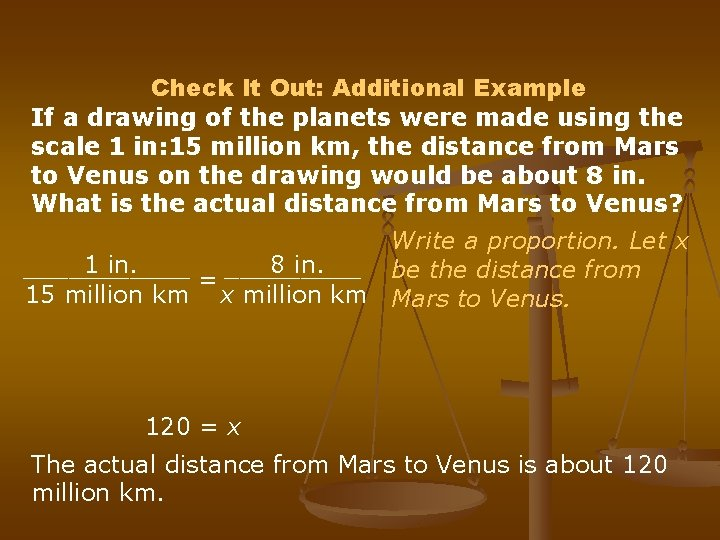Check It Out: Additional Example If a drawing of the planets were made using the scale 1 in: 15 million km, the distance from Mars to Venus on the drawing would be about 8 in. What is the actual distance from Mars to Venus? Write a proportion. Let x 1 in. 8 in. ______ be the distance from = 15 million km x million km Mars to Venus. 120 = x The actual distance from Mars to Venus is about 120 million km.Lesson Review: Try These! On a map of the Great Lakes area, 2 cm = 45 km. Find the actual distance of the following, given their distances on the map. 1. Detroit to Cleveland = 12 cm 2. Duluth to Nipigon = 20 cm 3. Buffalo to Syracuse = 10 cm 4. Sault Ste. Marie to Toronto = 33 cm 5. A state park is being added to this map. If the park is 450 km from Detroit, how far apart are these places on the map?